# Terms and expressions for specifying torque transducers

## Accuracy class

The accuracy class declared for HBM torque transducers means that the maximum of those deviations specified as percentages is equal to or less than the value declared as the accuracy class. The sensitivity tolerance is not included.

The accuracy class includes the following metrological properties explained in detail below:

• Linearity deviation including hysteresis (dlh)
• Relative standard deviation of repeatability (σrel)
• Temperature effect (per 10 K) on the zero signal (TK0)
• Temperature effect (per 10 K) on the sensitivity (TKc)

In transducers with two or more electrical outputs (frequency output and voltage output) the output with the highest accuracy is the deciding factor in determining the accuracy class. The accuracy class must not be mistaken for classification according to DIN 51309 or EA-10/14.

### Accuracy class vs. overall accuracy

The accuracy class provides practical guidance on the respective type series’ grouping within the HBM range of products. It must not be mistaken for the overall accuracy in practical use, with different individual influences acting at the same time.

#### Example:

We look at two versions of the T10F torque flange: on the one hand, option "S" (standard version) and on the other hand, option "G" (that is, reduced linearity deviation incl. hysteresis) in each case for the measuring ranges from 100 N·m to 10 kN·m.

In the data sheet the following maximum values are specified for the "S" version: 0.05 % for the temperature effect on the zero signal (TK0), 0.1 % for the temperature effect on sensitivity (TKC), and ±0.1 % for the linearity deviation incl. hysteresis (dlh). Due to the two values given last, the accuracy class is specified as 0.1. Version "G", however, offers an improved linearity deviation incl. hysteresis (dlh) amounting to 0.05 % only.

The temperature effect on sensitivity (TKC) is still 0.1 % and thus is the maximum deviation among those given as percentages. Therefore, the accuracy class for version "G" still has to be specified as 0.1.

Apparently, version "G" does not provide any increased benefit. However, it shows the relatively biggest effect only with regard to one characteristic value, the TKC. In addition, this characteristic value is the only measure for deviations related to the actual value. As a consequence, its influence is considerably smaller, for example, with measurements in the partial load range.

## Sensitivity C

The span between the output signal values at nominal torque and at zero torque. Usually two separate sensitivities are specified for HBM torque transducers, one for clockwise torque and one for counterclockwise torque.Fig. 1: Sensitivity and nominal torque

The sensitivity C characterizes the slope of the characteristic curve. The characteristic curve is chosen as the straight line connecting the output signal SM0 determined with mounted but unloaded torque transducer (initial torque signal) and the output signal Sn at nominal torque determined at increasing torque. This gives the simple equation

C = Sn − SM0

The sensitivity and the nominal torque form a known pair of values combining a given torque and the respective span of the output signal. If two such pairs of values are given they can be used for setting the amplifier. Usually, the second pair of values is zero torque and zero output signal span (i.e. output signal = initial torque signal).

### Nominal sensitivity

The nominal value characterizing the transducer’s sensitivity. Usually it is equal for clockwise and counterclockwise torque.

The nominal sensitivity is a value characterizing the respective transducer’s type and measuring range. However, the actual sensitivity of the individual is equal to the nominal sensitivity only within specified tolerances.

### Sensitivity tolerance

The permissible deviation of the actual sensitivity from the nominal sensitivity. It is given as a percentage with respect to the nominal sensitivity.

For HBM torque transducers the actual sensitivity of the individual is determined before delivery. The value is documented in the test certificate or calibration certificate. For this reason the sensitivity tolerance is not taken into consideration when determining the accuracy class.

## Temperature effect

### Temperature effect on the sensitivity

The temperature effect on the sensitivity is the variation of the actual output signal due to a 10 K change in temperature determined at nominal torque and related to the sensitivity. The specified value is the maximum occurring in the nominal temperature range.

The temperature effect on the sensitivity (also called the temperature coefficient of sensitivity) is a measure of the temperature effect on the output signal with a load applied to the transducer. When determining this value, the output signal has to be corrected by subtracting the initial torque signal at the respective temperature. A stationary temperature state has to be established.

The significant temperature is the transducer temperature. A stationary temperature state as defined at HBM means that the maximum temperature variation in a 15-minute period does not exceed 0.1 K. The amount of the deviation is given as a percentage of the actual span of the output signal with the respective torque applied (in the event of loading with the nominal torque this is the sensitivity).

The temperature effect on sensitivity results in a change of slope of the characteristic curve (see Fig. 2). It is of particular importance when a transducer is operated at a temperature differing significantly from the reference temperature. For partial load ranges, however, it has very little effect because the resulting deviation acts always as a percentage of the actual output signal span.

Please note that normally the temperature effect on sensitivity and the temperature effect on the zero point (TK0) are superimposed on each other.

#### Example:

Consider a torque transducer with 1 kN⋅m nominal torque, let the temperature effect on the sensitivity be specified as TKC ≤ 0.1 %, the reference temperature as 23 °C and the nominal temperaturerange from +10 °C to +60 °C.

If the transducer is operated at a temperature of 33 °C (or 13 °C), the sensitivity deviation due to the temperature variation may amount to up to 0.1 %.

For a torque of 1 kN⋅m (nominal torque) this amounts to a deviation in the displayed value of 1 N⋅m. For a torque of 200 N⋅m, however, the deviation amounts to 0.2 N⋅m only, since the TKC is always a percentage deviation referring to the actual output signal span. This is due to the fact that the sensitivity is referred to as the measure of the slope of the straight line. Using the same transducer at 43 °C (20 K deviation from the nominal temperature) may result in a maximum deviation of up to 0.2 % in the worst case. This does not apply to usage at 3 °C, since this temperature is not within the nominal temperature range.

### Temperature effect on the zero signal

The temperature effect on the zero signal is the variation, due to a 10-K change in temperature, in the unloaded transducer’s output signal related to the nominal sensitivity. The specified value is the maximum occurring in the nominal temperature range.

The temperature effect on the zero signal (also called the temperature coefficient of the zero signal) is determined by measuring the variation due to a 10–K change in temperature in the unloaded transducer’s actual output signal at zero torque after re-establishment of a stationary temperature state. The significant temperature is the transducer temperature. A stationary temperature state as defined at HBM means that the maximum temperature variation in a 15-minute period does not exceed 0.1 K.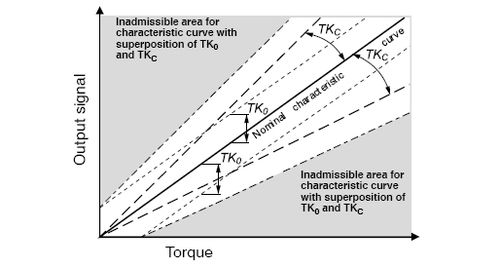Fig. 2: Temperature effect on the sensitivity TKC and on the zero point TK0.

The temperature effect on the zero signal results in a parallel shift in the characteristic curve (see Fig. 2). It is of particular importance when a transducer is operated at a temperature differing significantly from the reference temperature. By taring or zero balancing at operating temperature, the measurement error due to the temperature effect on the zero signal can be eliminated.

Please note that normally the temperature effect on the zero point and the temperature effect on the sensitivity (TKC) are superimposed on each other.

#### Example:

Consider a torque transducer with 1 kN⋅m nominal torque, let the temperature effect on the zero signal be specified as TK0 ≤ 0.05 %, the reference temperature as 23 °C and the nominal temperature range from +10 °C to +60 °C.

If the transducer is operated at a temperature of 33 °C (or 13 °C), the zero signal deviation may amount to up to 0.05 % of the nominal sensitivity. This corresponds to a deviation in the displayed value of 0.5 N⋅m. This deviation is independent of the torque with which the transducer is loaded.
Using the transducer at 43 °C may result in a maximum deviation of up to 0.1 % in the worst case.

This does not apply to usage at 3 °C, since this temperature is not within the nominal temperature range.

## Linearity deviation

Absolute value of the maximum deviation of a torque transducer’s characteristic curve determined with increasing load from the reference straight line which approximates the characteristic curve as a straight line. The specified value is expressed as a percentage of the sensitivity C.

For determining the linearity deviation, a series of measurements is taken with the load increasing from zero to the nominal torque. The reference straight line is the best-fit straight line through the initial point, such that the maximum deviations (upward/downward) from the measurement signal have the same amount (see Fig. 3). The specified linearity deviation is the maximum deviation of the actual output signal from the reference straight line. It can also be described as half the width of the tolerance band that is symmetrical about the reference straight line.

The linearity deviation has to be taken into consideration because usually when adjusting the measurement chain, a characteristic curve in the form of a straight line is assumed. It takes maximum effect when a transducer is used for a wide measuring range, in the most extreme case from zero torque up to nominal torque.Fig. 3: Determination of the linearity deviation

### Linearity deviation including hysteresis

The linearity deviation including hysteresis specifies the maximum deviation (according to absolute value) of the output signal value from the reference straight line. The reference straight line is the best-fit straight line through the starting point (see Fig. 5). Thus, both linearity deviation and hysteresis are taken into consideration. The specified value is expressed as a percentage of the sensitivity C.

The load cycle for determining the linearity deviation including hysteresis covers the loading of the transducer from zero up to nominal torque and the subsequent relieving down to zero torque (see Fig. 5).
The reference straight line is the best-fit straight line through the initial point, such that the maximum deviations (upward/downward) from the measurement signal have the same amount.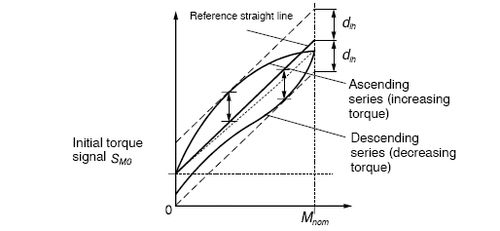Fig. 5 Determination of the linearity deviation including hysteresis dlh from a load-relieve cycle

The linearity deviation including hysteresis can also be interpreted as half the width of the tolerance band that is symmetrical about the reference straight line (see Fig. 5). The only difference to the procedure for the determination of the linearity deviation dlin is that the load cycle here includes also the measurements at decreasing torque. This difference takes effect on both the calculation of the reference straight line and the deviations from the reference straight line.

To determine this value, HBM proceeds as described below:

• The transducer is preloaded with counterclockwise torque in three load cycles from zero to 100 % of the nominal torque and back to zero torque. The purpose of this preloading is to eliminate the influence of mounting like settling of bolts and smoothing of contact surfaces
• One load cycle with counterclockwise torque and recording of the respective values for the measurement signal at the predefined load steps (when testing during production at HBM these steps are at torque 0 %, 50 %, 100 %, 50 % and 0 % of Mnom)
• The transducer is preloaded with clockwise torque in three load cycles from zero to 100 % of the nominal torque and back to zero torque
• One load cycle with clockwise torque and recording of the respective values for the measurement signal at the predefined torque steps
• The best-fit straight line is calculated according to the above definition, separately for clockwise torque and counterclockwise torque
• The amount of the maximum deviation from the best-fit straight line is determined separately for clockwise torque and counterclockwise torque

The linearity deviation including hysteresis is of importance because normally when adjusting the measurement chain, a characteristic curve in the form of a straight line is assumed. It takes maximum effect if a transducer is used for a wide measuring range and it is not relieved between two relevant measurements. The most extreme case is the use from zero torque up to nominal torque.

#### Example:

Consider aT10FS torque flange of which the maximum permissible linearity deviation including hysteresis is specified as dlh ≤ 0.05 % and the nominal sensitivity amounts to 5 kHz. Provided that the measurement chain has been adjusted optimally, the output signal error due to the linearity deviation and hysteresis may amount to 2.5 Hz at most.

## Relative reversibility error

The relative reversibility error is the difference of the output signals when measuring the same torque applied in increasing and decreasing steps (see Fig. 4). The specified value is the maximum deviation (according to absolute value) in the measuring range. It is specified as a percentage of the sensitivity C.

The relative reversibility error is a measure of hysteresis, that is, the difference between the characteristic curves determined with increasing and decreasing torque. For determining the relative reversibility error, a load cycle from zero torque through nominal torque and back is recorded. The practical calculation is based on measurements at a number of predefined points in the load cycle (e.g. 0 %, 50 %, 100 % of Mnom).

Hysteresis describes the dependency of the measuring signal on the transducer’s loading history. It is of particular importance if a transducer is used for a wide measuring range and no unloading takes place between acquiring two relevant measurement points. The most extreme case is the use from zero torque up to nominal torque. The effect of hysteresis occurring during a partial load cycle is usually significantly smaller than the hysteresis during a load cycle covering the entire nominal torque range.Fig. 4: Determination of the relative reversibility error dhy from a load-relieve cycle (here based on the load steps 0 %, 50 %, 100 % Mnom). The value to be specified is the maximum reversibility error of the given load steps (here dhy,0 and dhy,50)

## Relative Standard Deviation of Repeatability

Repeatability describes the property that the output signal is the same for all measurements of the same torque when measured several times. During the measurements the mounting position of the torque transducer shall remain unchanged and the torque transducer shall not be mounted and
dismounted (repeat conditions). The standard deviation indicates the average deviation between all measurements of the same torque when measured several times.

The relative standard deviation of repeatability is a measure of the repeatability according to DIN 1319. It is defined as the standard deviation of repeatability according to DIN 1319 and is expressed as a percentage of the range of the signal span covered during the testing procedure. It is a statistic measure of
random measurement deviations. For this reason, all those conditions are kept constant that, if changed, might cause variations in systematic measurement deviations (repeat conditions to DIN 1319).

The determination of the relative standard deviation of repeatability is a type test carried out on a static calibration system as follows:

• The torque transducer is preloaded up to nominal torque. The measurement signal S1,100% is taken at nominal torque
• The load is reduced to 50 % of the nominal load. The measurement signal S1,50% is taken at half the nominal torque
• Alternation between 50 % and 100 % of nominal torque. The measurement signals Si,50% and Si,100% are taken until 10 measured values each are available for each torque
• The equation below is used to calculate the relative standard deviation (in mathematical terminology, the empirical standard deviation of a random sample) for each torque and relate it to the output signal span: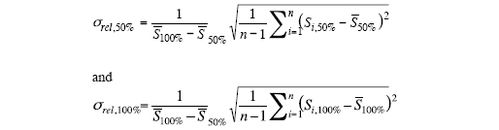with n=10 being the number of measurements for the respective torque applied (50 % or 100 %) and the arithmetic mean of the measurement signals according to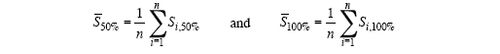• The technical data specifies the inferior of the two values σrel,50% and σrel,100% .

### Example:

HBM specifies the standard deviation of repeatability for its T10F torque transducer as σrel ≤ 0.03%. This value refers to the output signal span between the values of applied torque. Assuming a nominal torque of 1 kN·m, for example, this corresponds to a span of 500 N·m according to the test conditions specified above. Thus the standard deviation of repeatability in this case amounts to ≤ 0.15 N·m.

In the following example repeatability (quantitatively specified by the standard deviation of repeatability σrel) is of special importance to the user. A test bench for combustion engines is used for measurements with the aim of comparing different settings of the engine’s control electronics. The torque transducer’s mounting and the ambient conditions remain unchanged. The loading histories for the different measurement series are identical. In these measurements the differences between the torque values of the individual test series are more important than the absolute torque values. Under these circumstances, the contribution of the torque transducer to the uncertainty of these differences is given by its repeatability.

### Nominal rotation speed

The nominal rotation speed is the upper limit of the speed range starting from zero. It applies to both clockwise and counterclockwise rotation.

### Nominal torque

The nominal torque Mnom is the torque defining the upper limit of the range in which specified tolerances of the transducer properties are not exceeded.

### Maximum service torque

The maximum service torque is the upper limit of the range in which there is an unambiguous relation between output signal and torque. If within this range torque is increased above the nominal torque the limit values declared in the specifications may be exceeded.

If the transducer has been used between nominal torque and maximum service torque, the limit values given in the specifications will be kept by the transducer when it is used again for torque values up to nominal torque. A slight shift of the zero signal may occur, but this is not considered to be a violation of
the specifications.

The torque transducer can be used for measurements up to the maximum service torque, though the measurement properties may prove less favorable.

The limitation on maximum service torque may be given by electronic properties (such as the modulation range of the internal amplifier electronics) or by mechanical properties (such as an overload stop). In the case of transducers that have neither internal electronics nor mechanical overload protection, the maximum service torque and the limit torque are frequently identical.

### Limit torque

The limit torque is the torque up to which the transducer’s measuring capability will not suffer permanent damage.

If the transducer has been used between nominal torque and limit torque, the limit values given in the specifications will be kept by the transducer when it is used again for torque values up to nominal torque. A slight shift of the zero signal may occur, but this is not considered to be a violation of the specifications.

In the event of a continuous vibrating load, the limits discussed below in the section on permissible oscillation bandwidth have priority over the limit torque.

### Breaking torque

The breaking torque is the torque which when exceeded may lead to mechanical destruction of the transducer.

In the event of torque values between limit torque and breaking torque no mechanical destruction will occur, but the transducer may be damaged to such an extent that it will be permanently unusable.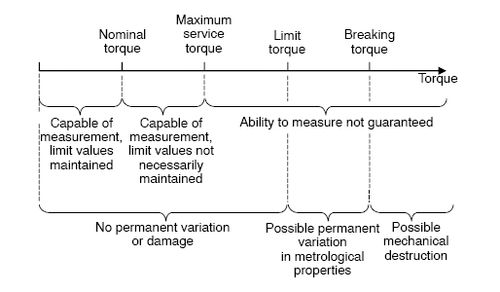## Permissible oscillation bandwidth

The permissible oscillation bandwidth is the oscillation amplitude of a sinusoidally varying torque with which the transducer can be stressed for 10⋅106 vibration cycles without causing any significant variations in its metrological properties.

The amplitude is specified as a peak-to-peak value, that is, as the difference between maximum and minimum torque. See also Fig. 7.

As well as the permissible vibration bandwidth it is also necessary to define a permissible upper limit for the torque which occurs. This upper limit usually coincides with the nominal torque (in both the positive and negative direction). Values which differ from this are explicitly declared in the specifications.

The concept has been taken from standard DIN 50100, which deals with continuous vibration testing (fatigue testing) within the context of materials testing, and has been transferred from mechanical stress to torque.

The deciding factor for fatigue strength is the number of vibration cycles alone. The frequency is not significant within the frequency range that is relevant to mechanical processes*). According to DIN 50100, it can be assumed to a close approximation for the case of steel materials that a mechanical component is fatigue proof under a given load if it endures the number of 10⋅106 load cycles under the respective load.

The upper limit for torque in the case of vibrational loading replaces explicit information about the mean vibrational loading. Within the range defined by the positive and negative limits, both pulsating torque and alternating torque are permissible (see Fig. 7).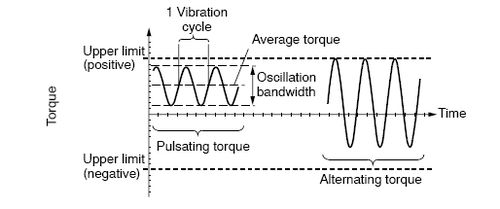Fig. 7: Terms used in connection with the oscillation bandwidth

----------

*) see also: H.-J. Bargel, G. Schulze: Werkstoffkunde (Materials Science), VDI-Verlag GmbH, Düsseldorf, Germany 1988

### Axial limit force

The axial limit force is the maximum permissible longitudinal force (or axial force), shown as Fa in Fig. 8. If the axial limit force is exceeded, the ability of the transducer to measure may be permanently damaged.

In HBM torque transducers the axial limit force sets an upper limit to the service range. Torque transducers can be used for measurement if the axial forces do not exceed the axial limit force. However, there may be some effect on the measurement signal. The upper limit for this influence is separately notified in the specifications.

The permissible axial force gets smaller than the specified axial limit force if another irregular stress occurs (such as a bending moment, lateral force or exceeding the nominal torque). Otherwise the limit values have to be reduced. If for example 30 % of both the bending limit moment and the lateral limit force occur, only 40 % of the axial limit force is allowed in the case that the nominal torque is not exceeded. If parasitic loads occur as continuous vibrating loads, the respective permissible vibration bandwidths may differ from the respective limit loads.

### Lateral limit force

The lateral limit force is the maximum permissible lateral force (in the case of radial force), shown as Fr in Fig. 8. If the lateral limit force is exceeded, the ability of the transducer to measure may be permanently damaged.

In HBM torque transducers the lateral limit force sets an upper limit to the service range. Torque transducers can be used for measurement if the lateral forces do not exceed the lateral limit force. However, there may be some effect on the measurement signal. The upper limit for this influence is separately notified in the specifications.

The permissible lateral force gets smaller than the specified lateral limit force if another irregular stress occurs (such as an axial force, bending moment or exceeding the nominal torque). Otherwise the limit values have to be reduced. If for example 30 % of both the axial limit force and the bending limit moment occur, only 40 % of the lateral limit force is allowed in the case that the nominal torque is not exceeded. If parasitic loads occur as continuous vibrating loads, the respective permissible vibration bandwidths may differ from the respective limit loads.

### Bending limit moment

The bending limit moment is the maximum permissible bending moment, shown as Mb in Fig. 8. If the bending limit moment is exceeded, the ability of the transducer to measure may be permanently damaged.

In HBM torque transducers the bending limit moment sets an upper limit to the service range. Torque transducers can be used for measurement if the bending moments do not exceed the bending limit moment. However, there may be some effect on the measurement signal. The upper limit for this influence is separately notified in the specifications.

The permissible bending moment gets smaller than the specified bending limit moment if another irregular stress occurs (such as an axial force, lateral force or exceeding the nominal torque). Otherwise the limit values have to be reduced. If for example 30 % of both the axial limit force and the lateral force limit occur, only 40 % of the bending limit moment is allowed in the case that the nominal torque is not exceeded. If parasitic loads occur as continuous vibrating loads, the respective permissible vibration bandwidths may differ from the respective limit loads.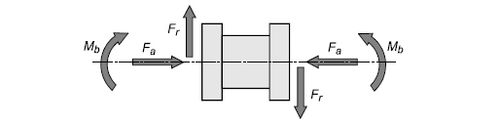Fig. 8: Parasitic loads - axial force Fa, lateral force Fr, bending moment Mb

## Temperatures

### Reference temperature

The reference temperature is the ambienttemperature at which the transducer specifications apply as far as there are no temperature ranges specifically defined in which the specifications concerned apply.

### Nominal temperature range

The nominal temperature range is the ambient temperature range within which the transducer can be operated for all practical purposes and within which it maintains the limit values for the metrological properties declared in the specifications.

### Service temperature range

The service temperature range is the ambient temperature range within which the transducer can be operated without permanent variations occurring in its metrological properties.

At temperatures within the service temperature range but outside the nominal temperature range, there is no guarantee that the limit values declared for the metrological properties in the specifications will be maintained.

### Storage temperature range

The storage temperature range is the ambient temperature range within which the transducer can be stored with no mechanical or electrical load without permanent variations occurring in its metrological properties.